# How to do a Correlation Graph in Excel- With Examples

When dealing with statistics, the major part of the bivariate analysis is the correlation of variables. Correlation can illustrate the relatedness of variables showing how close the relationship is. By using a correlation graph, you will be able to know if the relationship is

• Negative/ positive relationship
• Degree or strength of the linear relationship (whether the two variables have high degree/low or perfect strength)

With Excel, you can easily use scatter chart and trendline to create a correlation graph. The data is displayed as a collection of points where one variable is positioned o the vertical axis and the other on the horizontal axis.

## What is a Correlation Graph?

In simple terms, a correlation graph is a scatter plot that relies on coordinates in a 2D space to show values. A correlation graph is a comparison of two variables which are plotted on the X and Y variables.

In most cases, the value plotted on the X-axis is independent. This is because the dependent variable changes only if the independent value changes. The value plotted on the Y axis is also referred to as a measured or dependent variable.

Direction and interpretation of correlation graph

Positive and negative correlation

A positive relationship shows that when variable X increases, variable Y increases, and vice versa. For a negative relationship, it shows if variable X increases, variable Y will also decrease and vice versa. The following graphs can show these relationships.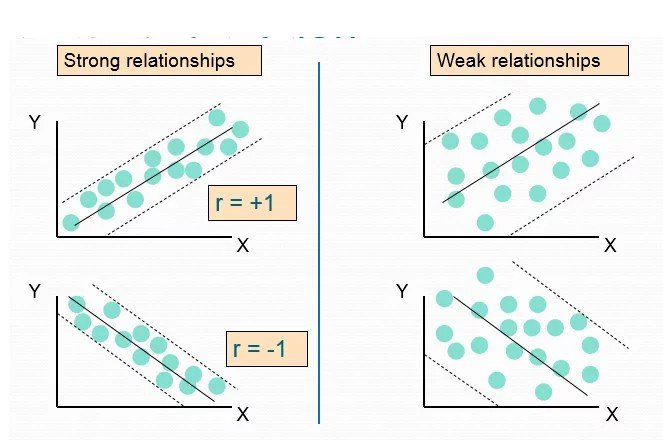No relationship

This graph shows that there is no relationship between the two variables. The following graph can show it.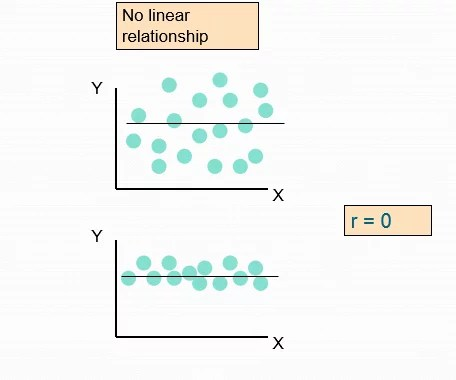How to do a correlation graph in Excel

You may be interested in measuring the relationship between the height and weight of a group of people. Here is how to go about it

1. The first step is creating the correlation data set. The independent variable is represented as the (X variable), and the dependent variable is represented as the (Y variable). Which should be on the left and right side, respectively. In this example, height is the independent variable, and weight is the dependent variable.2. Select all the original data tables in an open workbook.

3. Click on the Insert tab of the main menu ribbon.

4. In the Charts group, click on the Insert Scatter (X, Y) or Bubble Chart options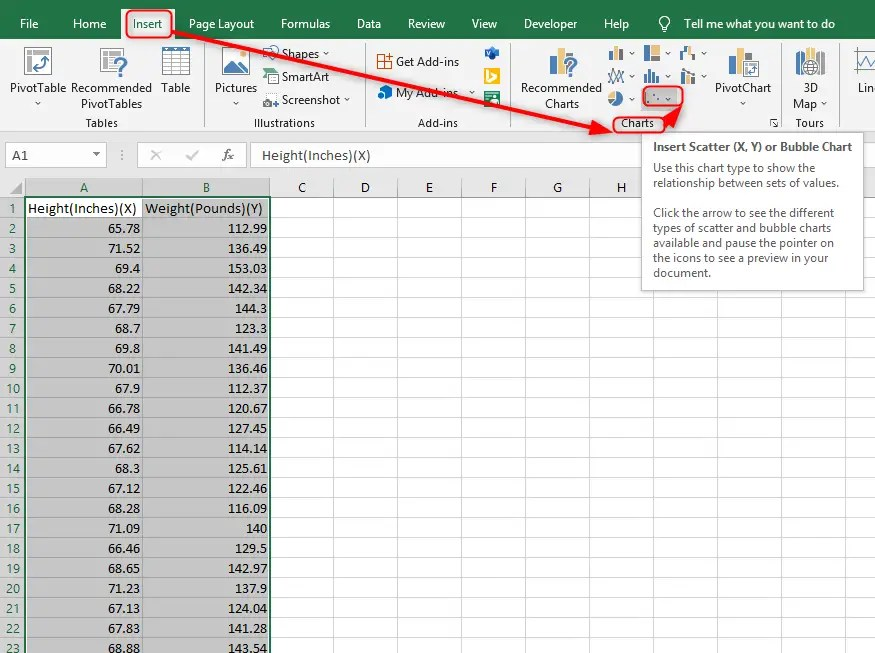5. A pop-up screen Scatter appears. Choose the Scatter part.6. There, you have your scatter plot.7. Name the chart a correlation chart (Height Vs. Weight) and name the X and Y axis. Select the Chart Title. Select the delete key and delete the existing Chart Title. You can now type the new text for the chart title (Height Vs. Weight).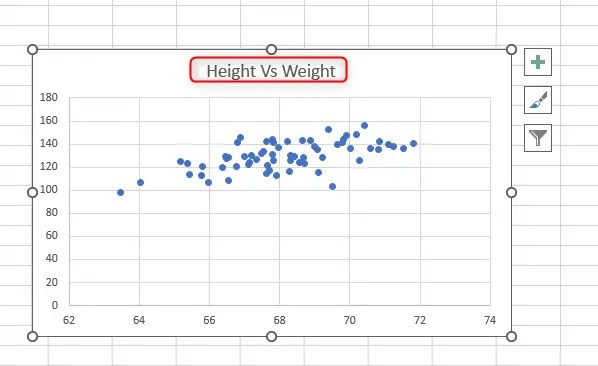8. For the Axis Title to do so, select the chart and from the top right corner, click on the “+” button (Chart Element) and then check the box of Axis Title.9. Edit the Axis titles to Height (Inches) for the X-axis and weight (pounds) for the y-axis. Do so by selecting the Axis Title. Select the delete key and delete the existing Axis Title. You can now type the new text for the Axis title.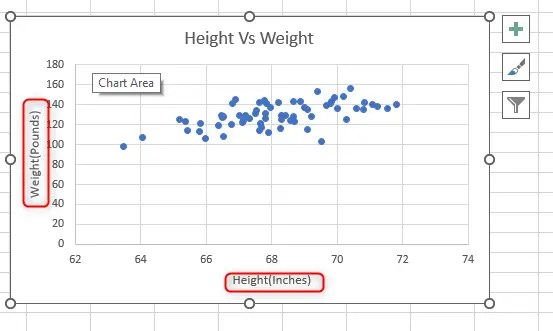10. Now add the trendline to show the correlation between height and weight. Select the chart, and from the top right corner, click on the “+” button (Chart Element) and then check the box of the trendline.11. You can format the trendline by Selecting the trendline and right-clicking. A pop-up menu appears to choose Format trendline. You can be able to set the intercept, Display the equation on the chart, or Display R- the squared value on the chart.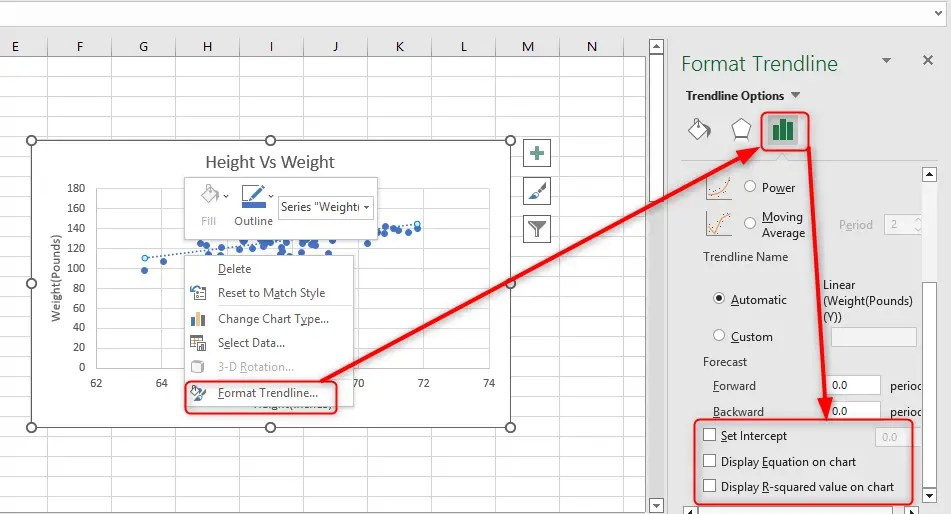12. Further, you can edit the trendline with the Fill & Line option.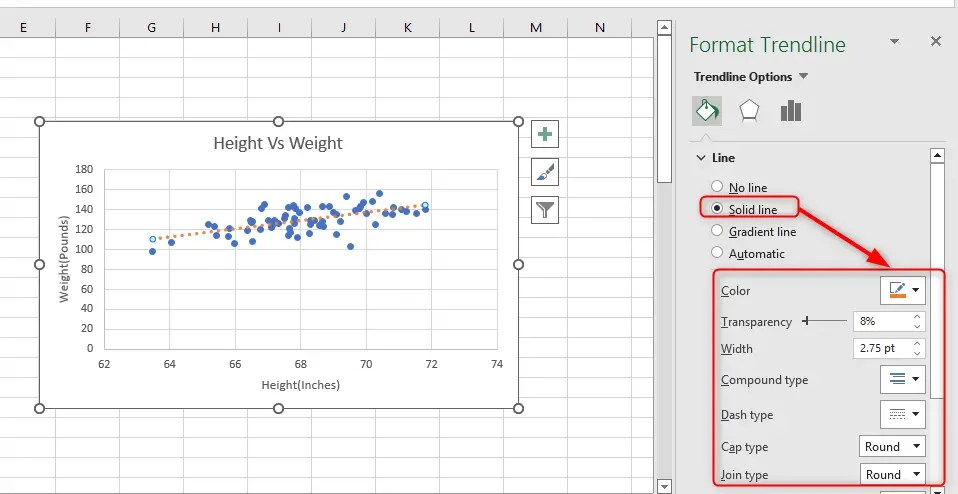## How to create a correlation graph in Excel Mobile App

The Excel mobile app comes in handy, especially when you want to access Excel on the go. It works on both Android and IOS devices. Whether you have a smartphone, a tablet, or an IPad, this app can be very useful. One way you might need to use the Mobile app is to create a correlation graph. Excel refers to the correlation graph as XY scatter. Follow these simple steps to create a correlation graph in the Excel Mobile app.

2. Enter the variables you wish to plot a graph for

3. Highlight all the values, including their titles

4. Click on the arrow button facing up in the bottom right corner.

5. Click on Home, then select insert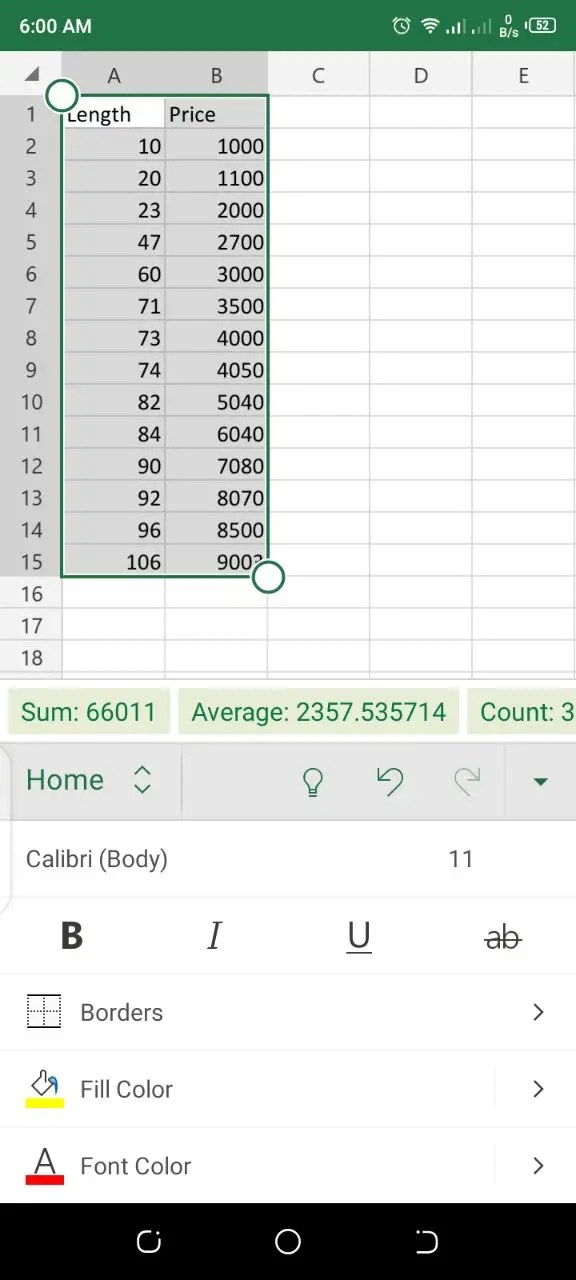6. Navigate to the chart options and click it.

7. Scroll down and select the X Y (Scatter) option

8. After selecting the XY scatter option, you will be prompted to choose a template. Choose any that suits your style.There you have it. You have successfully created a correction graph using the Excel mobile app.

## How to create a correlation graph on Google Sheets

Google sheets have an inbuilt feature that allows users to create a correlation graph easily. This section will guide you on how to create a correlation Graph on Google Sheets. Follow these simple steps

Step 1: Enter your values in two columns

Step 2: Highlight the values

Step 3: Click on the three dots at the top right corner.

Step 4: Select the chart icon from the provided list. Google sheets automatically pre-select a chart for you. You can change that in the chart editor>>chart type section if it’s not the one you wish to use. Here you need to choose a scatter plot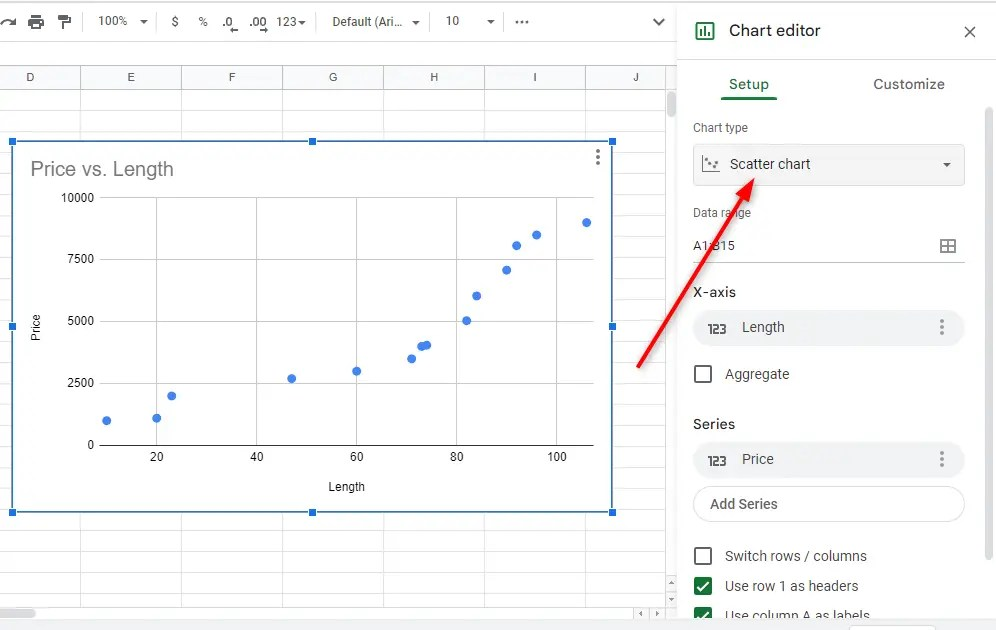### How to add a trend line to the correlation graph

A trendline is a straight line showcasing the data’s direction. It helps to give a clear picture of the correlation graph and its values. Follow these easy steps to add a trendline to your correlation graph.

1. Click on the three vertical buttons on the graph, then select edit chart to launch the chart editor.

2. Click the customize section and navigate to the series option. Expand this option

3. Scroll down a little bit and tick the trend line optionThis action will automatically create a line of best fit through the scattered values

4. You can change the line color by clicking the filled circle under the line color section

1. Which are the best graphs to show correlation?

The best graphs to show a correlation in Excel are line graphs and scatter graphs. These graphs can show a correlation between two given variables.

2. What is the best way to visualize correlation?

The best way to visualize correlation is by creating a scatter plot of the given variables.

3. Is a scatter diagram the same as a correlation Graph?

A scatter graph can also be referred to as a correlation graph, scatter plot or XY graph.

## Final Thoughts

As the name suggests, a correlation graph visually shows the relationship between two variables. Adding a trendline makes the interpretation of a scatter graph easier. A correlation graph is also referred to as an XY scatter graph Excel and Google sheets comes with inbuilt features that help uses to plot correlation graphs easily.